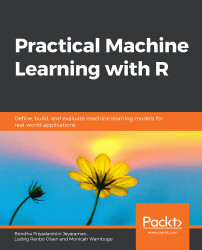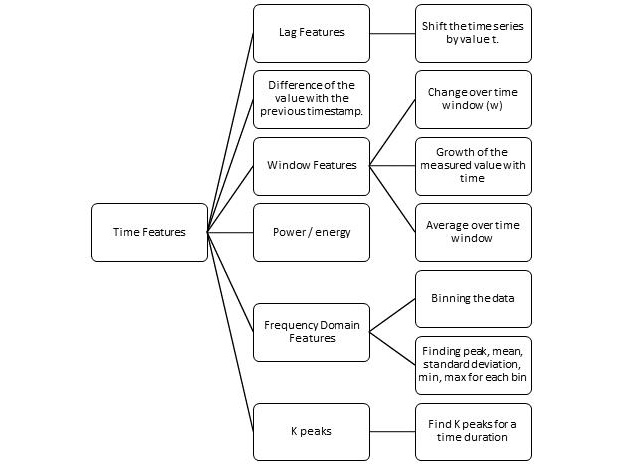•#### Practical Machine Learning with R#### Overview of this book

With huge amounts of data being generated every moment, businesses need applications that apply complex mathematical calculations to data repeatedly and at speed. With machine learning techniques and R, you can easily develop these kinds of applications in an efficient way. Practical Machine Learning with R begins by helping you grasp the basics of machine learning methods, while also highlighting how and why they work. You will understand how to get these algorithms to work in practice, rather than focusing on mathematical derivations. As you progress from one chapter to another, you will gain hands-on experience of building a machine learning solution in R. Next, using R packages such as rpart, random forest, and multiple imputation by chained equations (MICE), you will learn to implement algorithms including neural net classifier, decision trees, and linear and non-linear regression. As you progress through the book, you’ll delve into various machine learning techniques for both supervised and unsupervised learning approaches. In addition to this, you’ll gain insights into partitioning the datasets and mechanisms to evaluate the results from each model and be able to compare them. By the end of this book, you will have gained expertise in solving your business problems, starting by forming a good problem statement, selecting the most appropriate model to solve your problem, and then ensuring that you do not overtrain it.Free Chapter
An Introduction to Machine LearningData Cleaning and Pre-processingFeature EngineeringIntroduction to neuralnet and Evaluation MethodsLinear and Logistic Regression ModelsUnsupervised LearningAppendix## Time Series Features

Time series data is a special type of data where some quantities are measured over time, and therefore it contains data along with the timestamp. An examples would be stock prices and forecasting of the market, where we would have a stock name, stock value, and time as the time series data.

The following figure presents some time series features:###### Figure 3.3: Time series features

The time series features are as follows:

1. Lag features: Using the lag feature of time series data, we can shift the time series data values by a specific value.
2. Difference in value of timestamp: In time series data, it is important to derive the difference between timestamps.
3. Window Features: Window features are features that tend to change over a fixed interval of time (window). These could be measured using the change over time window, growth of measured value with time, or average over time window.
4. Power/Energy: This features denotes the power consumption...